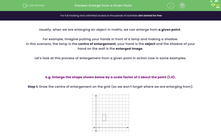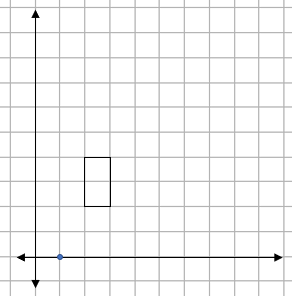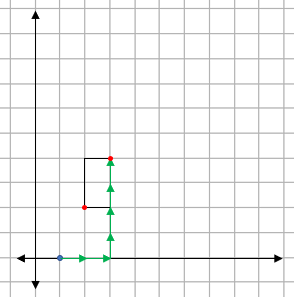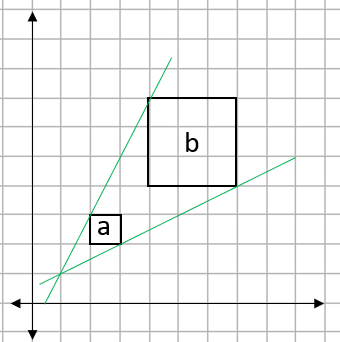# Enlarge from a Given Point

In this worksheet, students will enlarge shapes from a given point by applying a scale factor, as well as find the centre of enlargement and scale factor after an enlargement has occurred.Key stage:  KS 4

Year:  GCSE

GCSE Subjects:   Maths

GCSE Boards:   AQA, Eduqas, Pearson Edexcel, OCR,

Curriculum topic:   Geometry and Measures, Congruence and Similarity

Curriculum subtopic:   Properties and Constructions Plane Isometric Transformations

Difficulty level:#### Worksheet Overview

Usually, when we are enlarging an object in maths, we can enlarge from a given point

For example, imagine putting your hands in front of a lamp and making a shadow.

In this scenario, the lamp is the centre of enlargement, your hand is the object and the shadow of your hand on the wall is the enlarged image.

Let's look at this process of enlargement from a given point in action now in some examples.

e.g. Enlarge the shape shown below by a scale factor of 2 about the point (1,0).

Step 1: Draw the centre of enlargement on the grid (so we won't forget where we are enlarging from):Step 2: Find the movement from the centre to one of the points on the shape:We can see that to get to the bottom left corner of the rectangle from the centre, we need to move 1 square to the right and 2 up.

Step 3: Multiply this movement by the scale factor:

Our scale factor is 2, so this movement doubles to become 2 squares to the right and 4 up.

Step 4: Plot this point on our diagram (when we are doing this, it is vital to count from the centre of enlargement not the origin):Step 5: Repeat steps 2 - 4 for the other corners of the shape:Step 6: Draw the enlarged shape and label the image and enlargement:e.g. Shape a has been enlarged to become shape b. Find the scale factor and centre of enlargement.Finding the scale factor

We need to look closely at one of the pairs of sides.

If we use the horizontal base of each square, shape a is 1 square long and shape b is 3 squares long.

This means that our scale factor is 3.

Finding the centre of enlargement

To find this, we need to join up two pairs of matching corners with straight lines and see where they cross:We can see here that the two lines cross at the point (1,1), so this is our centre of enlargement.

In this activity, we will enlarge shapes from a given point by applying a scale factor, as well as find the centre of enlargement and scale factor after an enlargement has occurred.

You may find it helpful to have a pencil and some squared paper handy to support you in this activity. If you do not, you will need to be able to visualise these enlargements in your mind's eye.

### What is EdPlace?

We're your National Curriculum aligned online education content provider helping each child succeed in English, maths and science from year 1 to GCSE. With an EdPlace account you’ll be able to track and measure progress, helping each child achieve their best. We build confidence and attainment by personalising each child’s learning at a level that suits them.

Get started